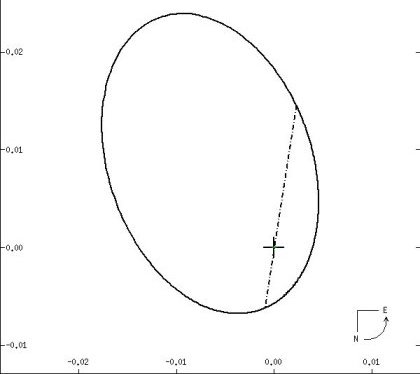THETA-2 TAU (Theta-2 Tauri). On the south side of the Hyades cluster of Taurus, the celestial Bull, in between the point of the classic "vee" that makes the Bull's head (marked by Gamma Tauri) and bright Aldebaran (Alpha Tau), lies a seeming naked-eye double, Theta Tauri, whose components are 337 seconds of arc (5.6 minutes, just under a tenth of a degree) apart. The third magnitude (3.40) eastern one, Theta- 2, is just a bit brighter than the fourth magnitude (3.84) component Theta-1 (if they are a real double at all; see below). Very near to us, direct parallax brings Theta-2 in at 150 light years (give or take 3), right in line with the mean distance of the Hyades of 152 light years. While Theta-2 and Theta-1 may or may not be a real pair, there is no doubt about the duplicity of Theta-2. The brighter, a class A (A7) "giant" (again see below), is paired with a star some three times fainter (also placing it somewhere in class A). The two are only a couple hundredths of a second of arc apart and orbit each other every 140.7 days.The companion to Theta-2 Tauri elliptically orbits its brighter mate (at the cross) every 140.7 days at a mean distance of 0.86 Astronomical Units. In reality, each orbits the other around a common center of mass. The orbit is tilted to the line of sight so that the major axis of the ellipse (the dashed line) appears off to the side and the focus of the true ellipse (at the cross) is offset as well. The scale on the axes is in hundredths of a second of arc. From the Sixth Catalog of Orbits of Visual Binary Stars , W. I. Hartkopf and B. D. Mason, US Naval Observatory Double Star Catalog, 2006.
With a measured temperature (actually from the combined light) of 7880 Kelvin, Theta-2 A, the brighter, shines at around 69 times that of the Sun, whereas the lesser, Theta-2 B, comes in at 19 solar, giving respective radii of 3.9 and 3.0 times that of the Sun and masses of 2.4 and 1.8 solar. A rotation speed of at least 78 km/s yields a rotation period for the brighter member of under 2.5 days. Theory also shows that Theta-2 A is more a subgiant (a star that has just given up core hydrogen fusion) than a true giant (such classification of mid-to-cool A stars very difficult). The age, estimated at 670 million years, falls very close to the 650 million year age of the cluster itself (as expected). Sophisticated interferometry gives a mean true separation between the two of 0.86 Astronomical Units (a high eccentricity taking the pair between 1.3 and 0.23 AU). Kepler's Laws then reveal masses of 2.4 and 1.9 times solar, right on the mark. A treasury of information and interest, Theta-2 Tau A makes its own mark as a distinctive "Delta Scuti" variable, a star that subtly oscillates in multiple modes. Twelve separate periods from 1.64 to 2.22 hours have been found for the star with variation sizes that range from 0.03 to 0.0005 magnitudes. Theta-1 formally lies at a distance of 154 light years, which would make physical bonding with Theta-2 impossible, but the errors are such that they could be at the same distance, making it at least marginally possible. If they ARE paired, they would still be at least 16,000 AU apart, and from the combined masses of both stars (Theta-1 also double), the orbital period would be at least 700,000 years. However, gravitational interactions among cluster stars would make such a scenario rather unlikely at best. (Thanks to Paolo Colona, who suggested this star.)